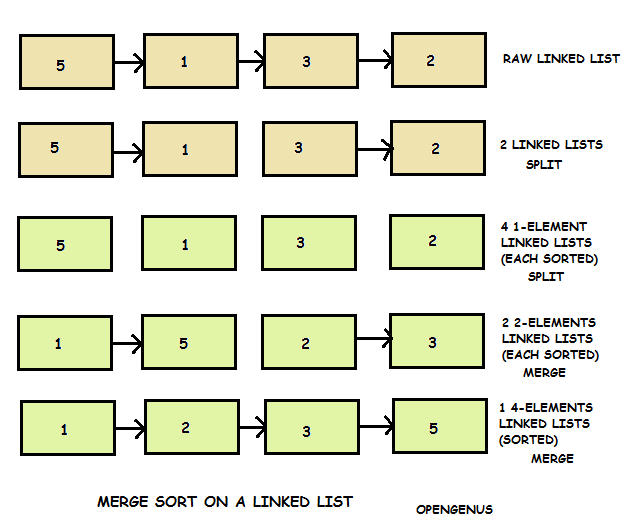×

Search anything:

# Merge Sort a singly Linked ListGet this book -> Problems on Array: For Interviews and Competitive Programming

Merge sort is a fast comparison based sorting algorithm which can be utilized in sorting a Linked List as well. Merge Sort is preferred for sorting a linked list. The slow random-access performance of a linked list makes some other algorithms such as Quick Sort perform poorly, and others such as Heap Sort completely impossible.

### Algorithm

The algorithm is same as that of Merge Sort will implementation changes to accomodate the structure of Linked List.### Pseudocode

``````

STEP 1: If head is NULL or there is only one element in the Linked List

STEP 2: Divide the linked list into two equal halves.

STEP 3: Sort the two halves first_half and second_half.
MergeSort(first_half);
MergeSort(second_half);

STEP 4: Merge the sorted first_half and second_half (using Merge_Sort() recursively)
``````

### Complexity

• Worst case time complexity: `Θ(N log N)`
• Average case time complexity: `Θ(N log N)`
• Best case time complexity: `Θ(N log N)`
• Space complexity: `Θ(1)`

### Implementations

Implementation of applying Merge Sort on a Linked List is as follows:

• C
• Java

### C

``````
#include<stdio.h>
#include<stdlib.h>
struct Node
{
int data;
struct Node* next;
};
struct Node* SortedMerge(struct Node* a, struct Node* b);
void FrontBackSplit(struct Node* source, struct Node** frontRef, struct Node** backRef);
{
struct Node* a;
struct Node* b;
{
return;
}
MergeSort(&a);
MergeSort(&b);
}
struct Node* SortedMerge(struct Node* a, struct Node* b)
{
struct Node* result = NULL;
if (a == NULL)
return(b);
else if (b==NULL)
return(a);
if (a->data <= b->data)
{
result = a;
result->next = SortedMerge(a->next, b);
}
else
{
result = b;
result->next = SortedMerge(a, b->next);
}
return(result);
}
void FrontBackSplit(struct Node* source, struct Node** frontRef, struct Node** backRef)
{
struct Node* fast;
struct Node* slow;
slow = source;
fast = source->next;
while (fast != NULL)
{
fast = fast->next;
if (fast != NULL)
{
slow = slow->next;
fast = fast->next;
}
}
* frontRef = source;
* backRef = slow->next;
slow->next = NULL;
}
void push(struct Node** head_ref, int new_data)
{
struct Node* new_node = (struct Node*) malloc(sizeof(struct Node));
new_node->data = new_data;
}
int main()
{
struct Node* res = NULL;
struct Node* a = NULL;
push(&a, 3);
push(&a, 1);
push(&a, 2);
MergeSort(&a);
getchar();
return 0;
}
``````

### Java

``````
{
static class node
{
int val;
node next;
public node(int val)
{
this.val = val;
}
}

node sortedMerge(node a, node b)
{
node result = null;
if (a == null)
return b;
if (b == null)
return a;

if (a.val &lt;= b.val)
{
result = a;
result.next = sortedMerge(a.next, b);
}
else
{
result = b;
result.next = sortedMerge(a, b.next);
}
return result;

}

node mergeSort(node h)
{
if (h == null || h.next == null)
{
return h;
}

node middle = getMiddle(h);
node nextofmiddle = middle.next;

middle.next = null;

node left = mergeSort(h);

node right = mergeSort(nextofmiddle);

node sortedlist = sortedMerge(left, right);
return sortedlist;
}

node getMiddle(node h)
{
//Base case
if (h == null)
return h;
node fastptr = h.next;
node slowptr = h;

while (fastptr != null)
{
fastptr = fastptr.next;
if(fastptr!=null)
{
slowptr = slowptr.next;
fastptr=fastptr.next;
}
}
return slowptr;
}

void push(int new_data)
{
node new_node = new node(new_data);
}

public static void main(String[] args)
{

li.push(3);
li.push(2);
li.push(1);

}

``````}
``````

### Questions

#### What is the time complexity of this algorithm if Linked List is already sorted?

O(N)
O(1)
O(N log N)
O(log N)#### OpenGenus Foundation

The official account of OpenGenus IQ backed by GitHub, DigitalOcean and Discourse

Improved & Reviewed by:

Merge Sort a singly Linked List